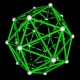# The Unapologetic Mathematician

## Classes of Manifolds

As we discussed the dimension of a manifold yesterday, we passed by an interesting construction that we want to look at in more detail.

Let$(U_1,\phi_1)$ and$(U_2,\phi_2)$ be two coordinate patches on an$n$-manifold$M$. We can restrict each coordinate map to the intersection of the two patches — if the intersection is empty this is trivial, but not wrong — and get a function from one open region of$\mathbb{R}^n$ to another. Specifically, if the coordinate patches have images$V_1=\phi_1(U_1\cap U_2)$ and$V_2=\phi_2(U_1\cap U_2)$, then we get a function$\phi_1\circ\phi_2^{-1}:V_2\to V_1$. We call this the “transition function” from one coordinate patch to another, and often write it$\phi_{2,1}$

So what do we know about these transition functions? Well,$\phi_{2,1}$ is a homeomorphism from$V_2$ to$V_1$, meaning it’s continuous with a continuous inverse. But remember that$V_i\subseteq\mathbb{R}^n$, and we know a lot about real$n$-dimensional space. We know all kinds of extra structure beyond just its topology, and in particular we know what it means for a function from$\mathbb{R}^n$ to itself to be smooth.

Now, as I’ve said before, “smooth” ends up behaving like a term of art. Really what we know is what it means for a function from$\mathbb{R}^n$ to itself to be differentiable, and for that differential to be continuous, and for there to be a second differential, and so on. We even introduced classes of functions to describe this whole tower, where$C^0$ consists of continuous functions,$C^1$ consists of continuously differentiable functions,$C^2$ consists of continuously twice-differentiable functions. This pattern continuous on through$C^k$ for continuously$k$-times-differentiable functions,$C^\infty$ for functions with arbitrarily many continuous derivatives, and$C^\omega$ for “analytic” functions which are the limits of their own Taylor series.

One case we didn’t explicitly mention is$PL$: the class of “piecewise linear” functions. These are continuous functions that can be defined by breaking up their domains into a finite number of chunks and giving an affine function — a linear transformation plus a constant offset vector — on each chunk so that the borders line up continuously. These functions aren’t generally differentiable, since they turn sharp corners at the boundaries of chunks, but they’re pretty nicely behaved anyway. The class$PL$ sits between$C^0$ and$C^1$.

Anyway, for each class of functions we can define a corresponding class of manifolds. If all the transition functions between all the coordinate patches are piecewise-linear, we say that we have a “PL-manifold”. If they’re all$C^1$, we say that we have a$C^1$-manifold or a “differential manifold”. This goes up through$C^k$-manifolds,$C^\infty$-manifolds, and analytic manifolds.

In practice, “smooth” ends up meaning$C^\infty$, so that we can always take as many derivatives as we want, but really we rarely need more than a few. Still, we’ll say “smooth” and not worry about it. And from here on all our manifolds will be smooth unless explicitly stated otherwise.

February 25, 2011 - Posted by | Differential Topology, Topology

## 10 Comments »

1. In “These functions aren’t generally continuous”, you meant “These functions aren’t generally differentiable”,right?Comment by Rafael | February 25, 2011 | Reply

• Ah, right. Thanks for catching me.Comment by John Armstrong | February 25, 2011 | Reply

2. […] Differentiable Structures It’s high time we introduced the “standard” smooth structures on real vector spaces, which are (of course) our models for all other smooth […]

Pingback by Standard Differentiable Structures « The Unapologetic Mathematician | March 2, 2011 | Reply

3. […] that we know what it means for coordinate patches to be compatible — for various definitions of “compatible” — we can define atlases on a […]

Pingback by Atlases and Structures « The Unapologetic Mathematician | March 2, 2011 | Reply

4. […] usual, we’re going to want our objects of study — smooth (or differentiable) manifolds — to be objects in a category. And a category means we need […]

Pingback by Smooth Maps « The Unapologetic Mathematician | March 2, 2011 | Reply

5. […] sheaves of use in differential topology. Given a smooth manifold — for whatever we choose smooth to mean — we can define sheaves of real algebras of real-valued functions for every […]

Pingback by Sheaves of Functions on Manifolds « The Unapologetic Mathematician | March 23, 2011 | Reply

6. […] all this means is that we take the transition function , take the th component, and take the th partial derivative of that function. And this is precisely […]

Pingback by Coordinate Transforms on Tangent Vectors « The Unapologetic Mathematician | April 1, 2011 | Reply

7. […] are the components of the Jacobian matrix of the transition function . What does this mean? Well, consider the linear […]

Pingback by Cotangent Vectors, Differentials, and the Cotangent Bundle « The Unapologetic Mathematician | April 13, 2011 | Reply

8. […] we can cancel off the two second partial derivatives because we’re assuming that is “smooth”, which in this case entails “has mixed second partial derivatives which commute” in any […]

Pingback by The Lie Bracket of Vector Fields « The Unapologetic Mathematician | June 2, 2011 | Reply

9. […] for smooth structures on and , we define them exactly as usual; real-valued functions on a patch of containing some […]

Pingback by Manifolds with Boundary « The Unapologetic Mathematician | September 13, 2011 | Reply